# A simple CNN for the MNIST dataset – VIII – filters and features – Python code to visualize patterns which activate a map strongly

Our series about a simple CNN trained on the MNIST data turns back to some coding.

In the last article I discussed an optimization algorithm which should be able to create images of pixel patterns which trigger selected feature maps of a CNN strongly. In this article we shall focus on the required code elements. I again want to emphasize that I apply and modify some basic ideas which I read in a book of F. Chollet and in a contribution of a guy called Mohamed to a discussion at kaggle.com (see my last article for references). A careful reader will notice differences not only with respect to coding; there are major differences regarding the normalization of intermediate data and the construction of input images. To motivate the latter point I first want to point out that OIPs are idealized technical abstractions and that not all maps may react to purely statistical data on short length scales.

# Images of OIPs which trigger feature maps are artificial technical abstractions!

In the last articles I made an explicit distinction between two types of patterns which we can analyze in the context of CNNs:

• FCP: A pattern which emerges within and across activation maps of a chosen (deep) convolutional layer due to filter operations which the CNN applied to a specific input image.
• OIP: A pattern which is present within the pixel value distribution of an input image and to which a CNN map reacts strongly.

Regarding OIPs there are some points which we should keep in mind:

• We do something artificial when we create an image of an elementary OIP pattern to which a chosen feature map reacts. Such an OIP is already an abstraction in the sense that it reflects an idealized pattern – i.e. a specific geometrical correlation between pixel values of an input image which passes a very specific filter combinations. We forget about all other figurative elements of the input image which may trigger other maps.
• There is an additional subtle point regarding our present approach to OIP-visualization:
Our algorithm – if it works – will lead to OIP images which trigger a
map’s neurons maximally on average. What does “average” mean with respect to the image area? A map always covers the whole input image area. Now let us assume that a filter combination of lower layers reacts to an elementary pattern limited in size and located somewhere on the input image. But some filters or filter combinations may be capable of detecting such a pattern at multiple locations of an input image.
One example would be a crossing of two relatively thin lines. Such a crossing could appear at many places in an input image. In fact, a trained CNN has seen several thousand images of handwritten “4”s where the crossing of the horizontal and the vertical line actually appeared at many different locations and may have learned about this when establishing filters. Under such conditions it is obvious that a map gets optimally activated if a relatively small elementary pattern appears multiple times within the image which our algorithm artificially constructs out of initial random data.
So our algorithm will with a high probability lead to OIP images which consist of a combination or a superposition of elementary patterns at multiple locations. In this sense an OIP image constructed due to the rule of a maximum average activation is another type of idealization. In a real MNIST image the re-occurrence of elementary patterns may not be present at all. Therefore, we should be careful and not directly associate the visualization of a pattern created by our algorithm with an elementary OIP or “feature”.
• The last argument can in some cases also be reverted: There may be unique large scale patterns which can only be detected by filters of higher (i.e. deeper) convolutional levels which filter coarsely and with respect to relatively large areas of the image. In our approach such unique patterns may only appear in OIP images constructed for maps of the deepest convolutional layer.

# Independence of the statistical data of the input image?

In the last article I showed you already some interesting patterns for certain maps which emerged from randomly varying pixel values in an input image. The fluctuations were forced into patterns by the discussed optimization loop. An example of the resulting evolution of the pixel values is shown below: With more and more steps of the optimization loop an OIP-pattern emerges out of the initial “chaos”.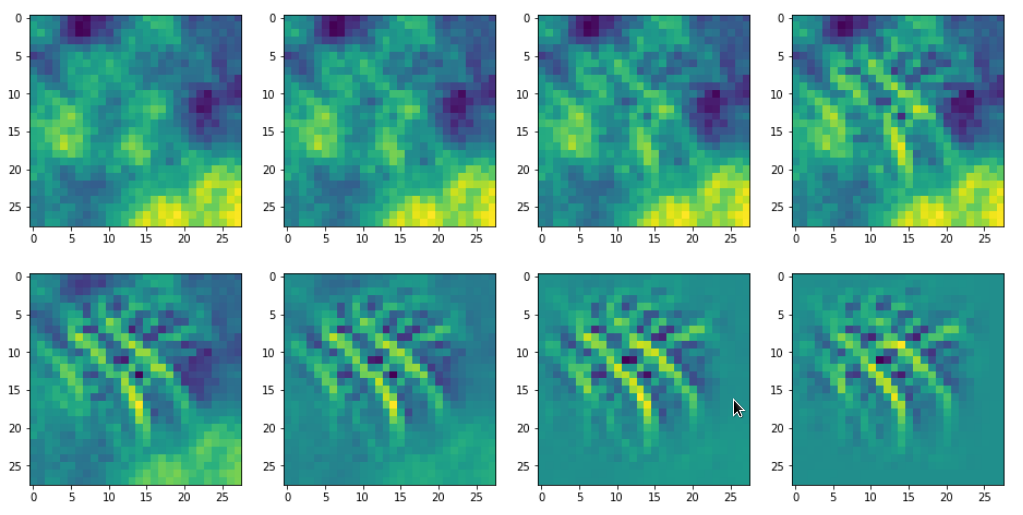Images were taken at optimization steps 0, 9, 18, 36, 72, 144, 288, 599 of a loop. Convergence is given by a change of the loss values between two different steps divided by the present loss value :
3.6/41 => 3.9/76 => 3.3/143 => 2.3/240 => 0.8/346 => 0.15/398 => 0.03/418

As we work with gradient ascent in OIP detection a lower loss means a lower activation of the map.

If we change the wavelength of the initial input fluctuations we get a somewhat, though not fundamentally different result (actually with a lower loss value of 381):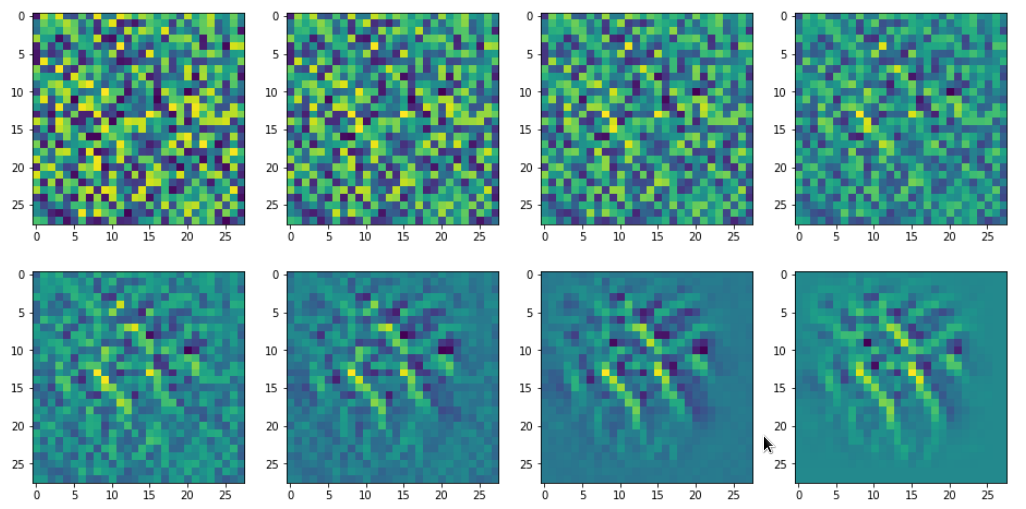This gives us confidence in the general usability of the method. However, I would like to point out that during your own experiments you may also experience the contrary:

For some maps and for some initial statistical input data varying at short length scales, only, the
optimization process will not converge. It will not even start to do so. Instead you may experience a zero activation of the selected map during all steps of the optimization for a given random input.

You should not be too surprised by this fact. Our CNN was optimized to react to patterns present in written digits. As digits have specific elements (features?) as straight lines, bows, circles, line-crossings, etc., we should expect that not all input will trigger the activation of a selected map which reacts on pixel value variations at relatively large length scales. Therefore, it is helpful to be able to vary the statistical input pattern at different length scales when you start your hunt for a nice visualization of an OIP and/or elementary feature.

All in all we cannot exclude a dependency on the statistical initial input image fluctuations. Our algorithm will find a maximum with respect to the specific input data fluctuations presented to him. Due to this point we should always be aware of the fact that the visualizations produced by our algorithm will probably mark a local maximum in the multidimensional parameter or representation space – not a global one. But also a local maximum may reveal significant sub-structures a specific filter combination is sensitive to.

# Libraries

To build a suitable code we need to import some libraries, which you first must install into your virtual Python environment:

```
import numpy as np
import scipy
import time
import sys
import math

from sklearn.preprocessing import StandardScaler
import tensorflow as tf
from tensorflow import keras as K
from tensorflow.python.keras import backend as B
from tensorflow.keras import models
from tensorflow.keras import layers
from tensorflow.keras import regularizers
from tensorflow.keras import optimizers
from tensorflow.keras.optimizers import schedules
from tensorflow.keras.utils import to_categorical
from tensorflow.keras.datasets import mnist

from tensorflow.python.client import device_lib

import matplotlib as mpl
from matplotlib import pyplot as plt
from matplotlib.colors import ListedColormap
import matplotlib.patches as mpat

import os
from os import path as path
```

# Basic code elements to construct OIP patterns from statistical input image data

To develop some code for OIP visualizations we follow the outline of steps discussed in the last article. To encapsulate the required functionality we set up a Python class. The following code fragment gives an overview about some variables which we are going to use. In the comments and explanations I sometimes used the word “reference” to mark a difference between

• addresses to some intermediate Tensorflow 2 [TF2] objects handled by a “watch()“-method of TF2’s GradientTape-object for eager calculation of gradients
• and eventual return objects (of a function based on keras’ backend) filled with values which we can directly use during the optimization iterations for corrections.

This is only a reminder of complex internal TF2 background operations based on complex layer models; I do not want to stress any difference in the sense of pointers and objects. Actually, I do not even know the precise programming patterns used behind TF2’s watch()-mechanism; but according to the documentation it basically records all operations involving the “watched” objects. The objective is the ability to produce gradient values of defined functions with respect to any variable changes instantaneously in eager execution later on.

```
# class to produce images of OIPs for a chosen CNN-map
# ****************************
***************************
class My_OIP:
'''
Version 0.2, 01.09.2020
~~~~~~~~~~~~~~~~~~~~~~~~~
This class allows for the creation and the display of OIP-patterns
to which a selected map of a CNN-model reacts

Functions:
~~~~~~~~~~
2) _build_oip_model()            => build an oip-model to create OIP-images
4) _oip_strat_0_optimization_loop():
=> Method implementing a simple strategy to create OIP-images
based on superposition of random data on large distance scales
5) _oip_strat_1_optimization_loop():
(NOT YET DEVELOPED)           => Method implementing a complex strategy to create OIP-images
based on partially evolved intermediate image
getting enriched by small scale fluctuations
6) _derive_OIP():                => Method used externally to start the creation of
an OIP for a chosen map
7) _build_initial_img_data()     => Method to construct an initial image based on
a superposition by random date on 4 different length scales

Requirements / Preconditions:
~~~~~~~~~~~~~~~~~~~~~~~~~~~~~
In the present version
* a CNN-model is assumed which works with standardized (!) input images,
* a CNN-Modell trained on MNIST data is assumed ,
* exactly 4 length scales for random data fluctations are used
to compose initial statistical image data
(roughly with a factor of 2 between them)

'''
def __init__(self, cnn_model_file = 'cnn_best.h5',
layer_name = 'Conv2D_3', map_index = 0,
img_dim = 28,
b_build_oip_model = True
):
'''
Input:
~~~~~~
cnn_model_file:     Name of a file containing  a full CNN-model
can later be overwritten by _load_cnn_model()
layer_name:         We can define a layer name we are interested in already when starting; s. below
map_index:          We may define a map we are interested in already when starting; s. below
img_dim:            The dimension of the assumed quadratic images

Major internal variables:
**************************
_cnn_model:             A reference to the CNN model object
_layer_name:            The name of convolutional layer
(can be overwritten by method _build_oip_model() )
_map_index:             index of the map in the layer's output array
(can later be overwritten by other methods)
_r_cnn_inputs:          A reference to the input tensor of the CNN model (here: 1 image - NOT a batch of images)
_layer_output:          Tensor with all maps of a certain layer

_oip_submodel:          A new model connecting the input of the cnn-model with a certain map

_tape:                  An instance of TF2's GradientTape-object
Watches input, output, loss of a model
and calculates gradients in TF2 eager mode
_r_oip_outputs:         A reference to the output of the new OIP-model
_r_oip_loss:            Reference to a loss
defined by operations on the OIP-output
_val_oip_loss:          Reference to a loss defined by operations on the OIP-output

_iterate:               Keras backend function to invoke the new OIP-model
and calculate both loss and gradient values (in TF2 eager mode)
This is the function to be used in the optimization loop for OIPs

Parameters controlling the optimization loop:
~~~~~~~~~~~~~~~~~~~~~~~~~~~~~~~~~~~~~~~~~~~
_oip_strategy:          0, 1 - There are two strategies to evolve OIP patterns out of statistical data - only the first strategy is supported in this version
0: Simple superposition of fluctuations at different length scales
1: Evolution over partially evolved images based on longer scale variations
enriched with fluctuations on shorter length scales
Both strategies can be combined with a precursor calculation

_b_oip_precursor:       True/False - Use a precursor analysis of long range variations
regarding loss => search for optimum variations for a given map
(Some initial input images do not trigger a map at all or
sub-optimally => We test out multiple initial fluctuation patterns).

_ay_epochs:             A list of 4 optimization epochs to be used whilst
evolving the img data via strategy 1 and intermediate images
_n_epochs:              Number of optimization epochs to be used with strategy 0

_n_steps:               Defines at how many intermediate points we show images and report
on the optimization process

_epsilon:               Factor to control the amount of correction imposed by
the gradient values of the OIP-model

Input image data of the OIP-model and references to it
~~~~~~~~~~~~~~~~~~~~~~~~~~~~~~~~~~
_initial_precursor_img  The initial image to start a precursor optimization with.
Would normally be an image of only long range fluctuations.
_precursor_image:       The evolved image updated during the precursor loop

_initial_inp_img_data:  A tensor representing the data of the input image
_inp_img_data:          A tensor representing the data of the input img during optimization
_img_dim:               We assume quadratic images to work with
with dimension _img_dim along each axis
For the time being we only support MNIST images

Parameters controlling the composition of random initial image data
~~~~~~~~~~~~~~~~~~~~~~~~~~~~~~~~~~~~~~~~~~~
_li_dim_steps:          A list of the intermediate dimensions for random data;
these data are smoothly scaled to the image dimensions
_ay_facts:              A Numpy array of 4 factors to control the amount of
contribution of the statistical variations
on the 4 length scales to the initial image

'''

# Input data and variable initializations
# ****************************************

# the model
self._cnn_model_file = cnn_model_file
self._
cnn_model      = None

# the layer
self._layer_name = layer_name
# the map
self._map_index  = map_index

# References to objects and the OIP sub-model
# ~~~~~~~~~~~~~~~~~~~~~~~~~~~~~~
self._r_cnn_inputs  = None # reference to input of the CNN_model
# also used in the OIP-model

self._layer_output  = None
self._oip_submodel  = None

self._tape          = None # TF2 GradientTape variable
# some "references"
self._r_oip_outputs = None # output of the OIP-submodel to be watched
self._r_oip_loss    = None
# respective values
self._val_oip_loss  = None

# The Keras function to produce concrete outputs of the new OIP-model
self._iterate       = None

# The strategy to produce an OIP pattern out of statistical input images
# ~~~~~~~~~~~~~~~~~~~~~~~~~--------~~~~~~
# 0: Simple superposition of fluctuations at different length scales
# 1: Move over 4 interediate images - partially optimized
self._oip_strategy = 0

# Parameters controlling the OIP-optimization process
# ~~~~~~~~~~~~~~~~~~~~~~~~~--------~~~~~~
# Use a precursor analysis ?
self._b_oip_precursor = False

# number of epochs for optimization strategy 1
self._ay_epochs    = np.array((20, 40, 80, 400))
len_epochs         = len(self._ay_epochs)

# number of epochs for optimization strategy 0
self._n_epochs     = self._ay_epochs[len_epochs-1]
self._n_steps      = 7   # divides the number of n_epochs into n_steps
# to produce intermediate outputs

# size of corrections by gradients
self._epsilon       = 0.01 # step-size for gradient correction

# Input images and references to it
# ~~~~~~~~
# precursor image
self._initial_precursor_img = None
self._precursor_img         = None
# The evetually used input image - a superposition of initial random fluctuations
self._initial_inp_img_data  = None  # The initial data constructed
self._inp_img_data          = None  # The data used and varied for optimization
# image dimension
self._img_dim               = img_dim   # = 28 => MNIST images for the time being

# Parameters controlling the setup of an initial image
# ~~~~~~~~~~~~~~~~~~~~~~~~~--------~~~~~~~~~~~~~~~~~~~
# The length scales for initial input fluctuations
self._li_dim_steps = ( (3, 3), (7,7), (14,14), (28,28) )
# Parameters for fluctuations  - used both in strategy 0 and strategy 1
self._ay_facts     = np.array( (0.5, 0.5, 0.5, 0.5) )

# ********************************************************
# Model setup - load the cnn-model and build the oip-model
# ************

if path.isfile(self._cnn_model_file):
# We trigger the initial load of a model
self._load_cnn_model(file_of_cnn_model = self._cnn_model_file, b_print_cnn_model = True)
# We trigger the build of a new sub-model based on the CNN model used for OIP search
self._build_oip_model(layer_name = self._layer_name, b_print_oip_model = True )
else:
print("<\nWarning: The standard file " + self._cnn_model_file +
" for the cnn-model could not be found!\n " +
a valid model")

return

```

The purpose of most of the variables will become clearer as we walk though the class’s methods below.

Let us say we have a trained CNN-model with all final weight parameters for node-connections saved in some h5-file (see the 4th article of this series for more info). We then can load the CNN-model and derive sub-models from its layer elements. The following method performs the loading task for us:

```
#
# Method to load a specific CNN model
# **********************************

# Check existence of the file
if not path.isfile(self._cnn_model_file):
print("<\nWarning: The file " + file_of_cnn_model +
" for the cnn-model could not be found!\n" +
"Please change the parameter \"file_of_cnn_model\"" +
" to load a valid model")

self._cnn_model_file = file_of_cnn_model

# Inform about the model and its file file 7
# ~~~~~~~~~~~~~~~~~~~~~~~~~~~
print("Used file to load a ´ model = ", self._cnn_model_file)
# we print out the models structure
if b_print_cnn_model:
self._cnn_model.summary()

# handle/references to the models input => more precise the input image
# ~~~~~~~~~~~~~~~~~~~~~~~~~~~~~~~~~~~~
#    Note: As we only have one image instead of a batch
#    we pick only the first tensor element!
#    The inputs will be needed for buildng the oip-model
self._r_cnn_inputs = self._cnn_model.inputs  # !!! We have a btach with just ONE image

# print out the shape - it should be known fro the original cnn-model
print("shape of cnn-model inputs = ", self._r_cnn_inputs.shape)

return

```

Actually, I used this function already in the class’ “__init__()”-method – provided the standard file for the last CNN exists. (In a more advanced version you would in addition check that the name of the standard CNN-model meets your expectations.)

The code is straightforward. You see that the structure of the original CNN-model is printed out if requested by the user.

Note also that we assigned the first element of the input tensor of the CNN-model, i.e. a single image tensor, to the variable “self._r_cnn_inputs”. This tensor will become a major ingredient in a new Keras model which we are going to build in a minute and which we shall use to calculate gradient components of a loss function with respect to all pixel values of the input image. The gradient’s component values will in turn be used during gradient ascent to correct the pixel values. Repeated corrections should lead to a systematic approach of a maximum of our loss function, which describes the map’s activation. (Remember: Such a maximum may depend on the input image fluctuations).

# Build a new Keras model based on the input tensor and a chosen layer

The next method is more interesting. We need to build a new Keras layer “model” based on the input layer and a deeper layer of the original CNN-model. (We already used the same kind of “trick” when we tried to visualize the activation output of a convolutional layer of the CNN.)

```
#
# Method to construct a model to optimize input for OIP-detection
#
***************************************
def _build_oip_model(self, layer_name = 'Conv2D_3', b_print_oip_model=True ):
'''
We need a Conv layer to build a working model for input image optimization.
We get the Conv layer by the layer's name.
The new model connects the first input element of the CNN to
the output maps of the named Conv layer CNN
'''
# free some RAM - hopefully
del self._oip_submodel

self._layer_name = layer_name
if self._cnn_model == None:
print("Error: cnn_model not yet defined.")
sys.exit()
# We build a new model based ion the model inputs and the output
self._layer_output = self._cnn_model.get_layer(self._layer_name).output

# We do not acre at the moment about the composition of the input
# We trust in that we handle only one image - and not a batch
model_name = "mod_oip__" + layer_name
self._oip_submodel = models.Model( [self._r_cnn_inputs], [self._layer_output], name = model_name)

# We print out the oip model structure
if b_print_oip_model:
print("Structure of the constructed OIP-sub-model:\n")
self._oip_submodel.summary()
return

```

We use the tensor for a single input image and the output of layer (= a collection of maps) of the original CNN-model as the definition elements of the new Keras model.

# TF2’s GradientTape() – watch the change of variables which have an impact on model gradients and the loss function

What do we have so far? We have defined a new Keras model connecting input and output data of a layer of the original model. TF2 can determine related gradients by the node connections defined in the original model. However, we cannot rely on a graph analysis by Tensorflow as we were used to with TF1. TF2 uses eager mode – i.e. it calculates gradients directly. What does “directly” mean? Well – as soon as changes to variables occur which have an impact on the gradient values. This in turn means that “something” has to watch out for such changes. TF2 offers a special object for this purpose: tf.GradientTape. See:
https://www.tensorflow.org/guide/eager

So, as a next step, we set up a method to take care of “GradientTape()”.

```    #
# *************************
'''
For TF2 eager execution we need to watch input changes and trigger gradient evaluation
'''
self._tape = None

# Watch out for input, output variables with respect to gradient chnages
# Input
# ~~~~~~~
self._tape.watch(self._r_cnn_inputs)
# Output
# ~~~~~~~
self._r_oip_outputs = self._oip_submodel(self._r_cnn_inputs)

# Loss
# ~~~~~~~
self._r_oip_loss = tf.reduce_mean(self._r_oip_outputs[0, :, :, self._map_index])
print(self._r_oip_loss)
print("shape of oip_loss = ", self._r_oip_loss.shape)

```

Note that the loss definition included in the code fragment is specific for a chosen map. This implies that we have to call this method every time we chose a different map for which we want to create OIP visualizations.

The advantage of the above code element is that “_tape()” can produce gradient values for the relation of the input data and loss data of a model automatically whenever we change the input image data. Gradient values can be called by

```  self._r_oip_grads  = self._tape.gradient(self._r_oip_loss, self._r_cnn_inputs)
```

As already discussed in my last article we apply a gradient ascent method to our “loss” function whose outcome rises with the activation of the neurons of a map. The following code sets up a method which first calls “_setup_gradient_tape()” and afterwards applies a normalization to the gradient values which “_tape()” produces. It then defines a convenience function and eventually calls a method which runs the optimization loop.

```    #
# Method to derive OIP from a given initial input image
# ********************
def _derive_OIP(self, map_index = 1, n_epochs = None, n_steps = 4,
epsilon = 0.01,
conv_criterion = 5.e-4,
b_stop_with_convergence = False ):
'''
V0.3, 31.08.2020
This method starts the process of producing an OIP of statistical input image data

Requirements:    An initial input image with statistical fluctuations of pixel values
-------------    must have been created.

Restrictions:    This version only supports the most simple strategy - "strategy 0":
-------------    Optimize in one loop - starting from a superposition of fluctuations
No precursor, no intermediate evolution of input image data

Input:
------
map_index:       We can chose a map here       (overwrites previous settings)
n_epochs:        Number of optimization steps  (overwrites previous settings)
n_steps:         Defines number of intervals (with length n_epochs/ n_steps) for reporting
This number also sets a requirement for providing n_step external axis-frames
to display intermediate images of the emerging OIP
=> see _oip_strat_0_optimization_loop()
epsilon:         Size for corrections by gradient values
conv_criterion:  A small threshold number for (difference of loss-values / present loss value )
b_stop_with_convergence:
Boolean which decides whether we stop a loop if the conv-criterion is fulfilled

'''
self._map_index = map_index
self._n_epochs  = n_epochs
self._n_steps   = n_steps
self._epsilon   = epsilon

# number of epochs for optimization strategy 0
# ~~~~~~~~~~~~~~~~~~~~~~~~~~~~~~~~~~~~~~~~~~~~~~
if n_epochs == None:
len_epochs = len(self._ay_epochs)
self._n_epochs   = self._ay_epochs[len_epochs-1]
else:
self._n_epochs = n_epochs

# Rest some variables
self._val_oip_loss  = None
self._iterate       = None

# Setup the TF2 GradientTape watch
# ~~~~~~~~~~~~~~~~~~~~~~~~~~~~~~~~~~~~~~~~~~~~~~~~~~~

# ~~~~~~~~~~~~~~~~~~

# define an abstract recallable Keras function
# producing loss and gradients for corrected img data
# the first list of addresses points to the input data, the last to the output data
self._iterate = B.function( [self._r_cnn_inputs], [self._r_oip_loss, self._r_oip_grads] )

# get the initial image into a variable for optimization
self._inp_img_data = None
self._inp_img_data = self._initial_inp_img_data

# Optimization loop for strategy 0
# ~~~~~~~~~~~~~~~~~~~~~~~~~~~~~~~~
if self._oip_strategy == 0:
self._oip_strat_0_optimization_loop( conv_criterion = conv_criterion,
b_stop_with_convergence = b_stop_with_convergence )

```

• We do not wildly accelerate our path on the loss hyperplane when some local pixel areas want to drive us into a certain direction.
• We reduce the chance of creating pixel values out of normal boundaries.

The last point fits well with a situation where the CNN has been trained on normalized or standardizes input images. Due to the normalization the size of pixel value corrections now depends significantly on the factor “epsilon”. We should choose it small enough with respect to the pixel values.

Another interesting statement in the above code fragment is

```        self._iterate = B.function( [self._r_cnn_inputs], [self._r_oip_loss, self._r_oip_grads] )
```

Here we use the Keras Backend to define a convenience function which relates input data with dependent outputs, whose calculations we previously defined by suitable statements. The list which is used as the first parameter of this function “_iterate()” defines the input variables, the list used as a second parameter defines the output variables which will internally be calculated via the GradientTape-functionality defined before. The “_iterate()”-function makes it much easier for us to build the optimization loop.

# The optimization loop for the construction of images that visualize OIPs and features

The optimization loop must systematically correct the pixel values to approach a maximum of the loss function. The following method “_oip_strat_0_optimization_loop()” does this job for us. (The “0” in the method’s name refers to a first simple approach.)

```    #
# Method to optimize an emerging OIP out of statistical input image data
# (simple strategy - just optimize, no precursor, no intermediate pattern evolution
# ********************************
def _oip_strat_0_optimization_loop(self, conv_criterion = 5.e-4,
b_stop_with_
convergence = False ):

'''
V 0.2, 28.08.2020

Purpose:
This method controls the optimization loop for OIP reconstruction of an initial
input image with a statistical distribution of pixel values.
It also provides intermediate output in the form of printed data and images.

Parameter:
conv_criterion:  A small threshold number for (difference of loss-values / present loss value )
b_stop_with_convergence:
Booelan which decides whether we stop a loop if the conv-criterion is fulfilled

This method produces some intermediate output during the optimization loop in form of images.
It uses an external grid of plot frames and their axes-objects. The addresses of the
axis-objects must provided by an external list "li_axa[]".
We need a seqence of >= n_steps axis-frames length(li_axa) >= n_steps).
With Jupyter the grid for plots can externally be provided by statements like

# figure
# -----------
#sizing
fig_size = plt.rcParams["figure.figsize"]
fig_size = 16
fig_size = 8
fig_a = plt.figure()
li_axa = [axa_1, axa_2, axa_3, axa_4, axa_5, axa_6, axa_7, axa_8]

'''

# Check that we really have an input image tensor
if ( (self._inp_img_data == None) or
(self._inp_img_data.shape != self._img_dim) or
(self._inp_img_data.shape != self._img_dim) ) :
print("There is no initial input image or it does not fit dimension requirements (28,28)")
sys.exit()

# Print some information
print("*************\nStart of optimization loop\n*************")
print("Strategy: Simple initial mixture of long and short range variations")
print("Number of epochs = ", self._n_epochs)
print("Epsilon =  ", self._epsilon)
print("*************")

# some initial value
loss_old = 0.0

# Preparation of intermediate reporting / img printing
# --------------------------------------
# Number of intermediate reporting points during the loop
steps = math.ceil(self._n_epochs / self._n_steps )
# Logarithmic spacing of steps (most things happen initially)
n_el = math.floor(self._n_epochs / 2**(self._n_steps) )
li_int = []
for j in range(self._n_steps):
li_int.append(n_el*2**j)
print("li_int = ", li_int)
# A counter for intermediate reporting
n_rep = 0
# Array for intermediate image data
li_imgs = np.zeros((self._img_dim, self._img_dim, 1), dtype=np.float32)

# Convergence? - A list for steps meeting the convergence criterion
# ~~~~~~~~~~~~
li_conv = []

# optimization loop
# *******************
# A counter for steps with zero loss and gradient values
n_zeros = 0

for j in range(self._n_epochs):

# Get output values of our Keras iteration function
# ~~~~~~~~~~~~~~~~~~~

# loss difference to last step - shuold steadily become smaller
loss_diff = self._
val_oip_loss - loss_old
#print("loss_val = ", loss_val)
#print("loss_diff = ", loss_diff)
loss_old = self._val_oip_loss

if j > 10 and (loss_diff/(self._val_oip_loss + 1.-7)) < conv_criterion:
li_conv.append(j)
lenc = len(li_conv)
# print("conv - step = ", j)
# stop only after the criterion has been met in 4 successive steps
if b_stop_with_convergence and lenc > 5 and li_conv[-4] == j-4:
return

# Check if our map reacts at all
# ~~~~~~~~~~~~~~~~~~~~~~~~~~~~~~~~
if self._val_oip_loss == 0.0 and avg_grads_val == 0.0:
print( "0-values, j= ", j,
" loss = ", self._val_oip_loss, " avg_loss = ", avg_grads_val )
n_zeros += 1

if n_zeros > 10:
print("More than 10 times zero values - Try a different initial random distribution of pixel values")
return

# gradient ascent => Correction of the input image data
# ~~~~~~~~~~~~~~~

# Standardize the corrected image - we won't get a convergence otherwise
# ~~~~~~~~~~~~~~~~~~~~~~~~~~~~~~~
self._inp_img_data = tf.image.per_image_standardization(self._inp_img_data)

# Some output at intermediate points
#     Note: We us logarithmic intervals because most changes
#           appear in the intial third of the loop's range
if (j == 0) or (j in li_int) or (j == self._n_epochs-1) :
# print some info
print("\nstep " + str(j) + " finalized")
print("present loss_val = ", self._val_oip_loss)
print("loss_diff = ", loss_diff)
# display the intermediate image data in an external grid
imgn = self._inp_img_data[0,:,:,0].numpy()
#print("Shape of intermediate img = ", imgn.shape)
li_axa[n_rep].imshow(imgn, cmap=plt.cm.viridis)
# counter
n_rep += 1

return

```

The code is easy to understand: We use our convenience function “self._iterate()” to produce actual loss and gradient values within the loop. Then we change the pixel values of our input image and feed the changed image back into the loop. Note the positive sign of the correction! All complicated gradient calculations are automatically and “eagerly” done internally thanks to “GradientTape”.

We said above that we limited the gradient values. How big is the size of the resulting corrections compared to the image data? If and when we use standardized image data and scale our gradient to unit length size then the relative size of the changes are of the order of the step size “epsilon” for the correction. In our case we set epsilon to 5.e-4.

The careful reader has noticed that I standardized the image data after the correction with the (normalized) gradient. Well, this step proved to be absolutely necessary to get convergence in the sense that we really approach an extremum of the cost function. Reasons are:

• My CNN was trained on standardized MNIST input images.
• We did not include any normalization layers into our CNN.
• Without counter-measures our normalized gradient values would eventually drive unlimited activation
values.

The last point deserves some explanation:
We used the ReLU-function as the activation function of nodes in the inner layers of our CNN. For positive input values ReLU actually is a linear function. Now let us assume that we have found a rather optimal input pattern which via a succession of basically linear operations drives an activation pattern of a map’s neurons. What happens if we just add constant small values to each pixel per iteration step? The output values after the sequence of linear transformations will just grow! With our method and the ReLU activation function we walk around a surface until we reach a linear ramp and climb it up. Without compensatory steps we will not find a real maximum because there is none. The situation is very different at the convolutional layers than at the eventual output layer of the CNN’s MLP-part.

You may ask yourself why we experienced nothing of this during the classification training? First answer: We did not optimize input data but weights during the training. Second answer: During training we did NOT maximize potentially unbound activation values but minimized a cost function based on output values of the last a MLP-layer. And these values were produced by a sigmoid function! The sigmoid function limits any input to the range ]0, +1[. In addition, the cost function (categorial_crossentropy) is designed to be convex for deviations of limited calculated values from a limited target vector.

The consequence is that we have to limit the values of the (corrected) input data and the related gradients in our present optimization procedure at the same time! This is done by the standardization of the image data. Remember that the correction values are around of the relative order of 5.e-4. In the end this is the order of the fluctuations which are unavoidable in the final OIP image; but now we have a chance to converge to a related small region around a real maximum.

The last block in the code deals with intermediate output – not only printed data on the loss function but also in form of intermediate images of the hopefully emerging pattern. These images can be provided in an external grid of figures in e.g. a Jupyter environment. The important point is that we define a suitable number of Matplotlib’s axis-objects and deliver their addresses via an external array “li_axa[]”. I am well aware of that the plotting solution coded here is a very basic one and it requires some ahead planning of the user – feel free to program it in a better way.

# Initial input image data – with variations on different length scales

We lack just one further ingredient: We need a method to construct an input image with statistical data. I have discussed already that it may be helpful to vary data on different length scales. A very simple approach to tackle this problem manually is the following one:

We use squares – each with a different small and limited number of cells; e.g. a (4×4)-, a (7×7)-, a (14×14)- and (28×28)-square. Note that (28×28) is the original size of the MNIST images. For other samples we may need different sizes of such squares and more of them. We then fill the cells with random numbers between [-1, 1]. We smoothly scale the variations of the smaller squares up to the real input image size; here: (28×28). We can do this by applying a bicubic interpolation to the data. In the end we add up all random data and normalize or standardize the resulting distribution of pixel values. See the code below for details:

```    #
# Method to build an initial image from a superposition of random data on different length scales
# ***********************************
def _build_initial_img_data( self,
strategy = 0,
li_epochs    = (20, 50, 100, 400),
li_
facts     = (0.5, 0.5, 0.5, 0.5),
li_dim_steps = ( (3,3), (7,7), (14,14), (28,28) ),
b_smoothing = False):

'''
V0.2, 31.08.2020
Purpose:
~~~~~~~~
This method constructs an initial input image with a statistical distribution of pixel-values.
We use 4 length scales to mix fluctuations with different "wave-length" by a simple
approach:
We fill four squares with a different number of cells below the number of pixels
in each dimension of the real input image; e.g. (4x4), (7x7, (14x14), (28,28) <= (28,28).
We fill the cells with random numbers in [-1.0, 1.]. We smootly scale the resulting pattern
up to (28,28) (or what ever the input image dimensions are) by bicubic interpolations
and eventually add up all values. As a final step we standardize the pixel value distribution.

Limitations
~~~~~~~~~~~
This version works with 4 length scales. it only supports a simple strategy for
evolving OIP patterns.
'''

self._oip_strategy = strategy
self._ay_facts     = np.array(li_facts)
self._ay_epochs    = np.array(li_epochs)

self._li_dim_steps = li_dim_steps

fluct_data = None

# Strategy 0: Simple superposition of random patterns at 4 different wave-length
# ~~~~~~~~~~
if self._oip_strategy == 0:

dim_1_1 = self._li_dim_steps
dim_1_2 = self._li_dim_steps
dim_2_1 = self._li_dim_steps
dim_2_2 = self._li_dim_steps
dim_3_1 = self._li_dim_steps
dim_3_2 = self._li_dim_steps
dim_4_1 = self._li_dim_steps
dim_4_2 = self._li_dim_steps

fact1 = self._ay_facts
fact2 = self._ay_facts
fact3 = self._ay_facts
fact4 = self._ay_facts

# print some parameter information
# ~~~~~~~~~~~~~~~~~~~~~~~~~~~~~~~~
print("\nInitial image composition - strategy 0:\n Superposition of 4 different wavelength patterns")
print("Parameters:\n",
fact1, " => (" + str(dim_1_1) +", " + str(dim_1_2) + ") :: ",
fact2, " => (" + str(dim_2_1) +", " + str(dim_2_2) + ") :: ",
fact3, " => (" + str(dim_3_1) +", " + str(dim_3_2) + ") :: ",
fact4, " => (" + str(dim_4_1) +", " + str(dim_4_2) + ")"
)

# fluctuations
fluct1 =  2.0 * ( np.random.random((1, dim_1_1, dim_1_2, 1)) - 0.5 )
fluct2 =  2.0 * ( np.random.random((1, dim_2_1, dim_2_2, 1)) - 0.5 )
fluct3 =  2.0 * ( np.random.random((1, dim_3_1, dim_3_2, 1)) - 0.5 )
fluct4 =  2.0 * ( np.random.random((1, dim_4_1, dim_4_2, 1)) - 0.5 )

# Scaling with bicubic interpolation to the required image size
fluct1_scale = tf.image.resize(fluct1, [28,28], method="bicubic", antialias=True)
fluct2_scale = tf.image.resize(fluct2, [28,28], method="bicubic", antialias=True)
fluct3_scale = tf.image.resize(fluct3, [28,28], method="bicubic", antialias=True)
fluct4_scale = fluct4

# superposition
fluct_data = fact1*fluct1_scale + fact2*fluct2_scale + fact3*fluct3_scale + fact4*fluct4_scale

# get the standardized plus smoothed and unsmoothed image
# ~~~~~~~~~~~~~~~~~~~~~~~~~~~~~~~~~~~~~~~~
#    TF2 provides a function performing standardization of image data function
r
fluct_data_unsmoothed = tf.image.per_image_standardization(fluct_data)
fluct_data_smoothed   = tf.image.per_image_standardization(
tf.image.resize( fluct_data, [28,28],
method="bicubic", antialias=True) )

if b_smoothing:
self._initial_inp_img_data = fluct_data_smoothed
else:
self._initial_inp_img_data = fluct_data_unsmoothed

# There should be no difference
img_init_unsmoothed = fluct_data_unsmoothed[0,:,:,0].numpy()
img_init_smoothed   = fluct_data_smoothed[0,:,:,0].numpy()

ax1_1.imshow(img_init_unsmoothed, cmap=plt.cm.viridis)
ax1_2.imshow(img_init_smoothed, cmap=plt.cm.viridis)

print("Initial images plotted")

return self._initial_inp_img_data

```

The factors fact1, fact2 and fact3 determine the relative amplitudes of the fluctuations at the different length scales. Thus the user is e.g. able to suppress short-scale fluctuations completely.

I only took exactly four squares to simulate fluctuation on different length scales. A better code would make the number of squares and length scales parameterizable. Or it would work with a Fourier series right from the start. I was too lazy for such elaborated things. The plots again require the definition of some external Matplotlib figures with axis-objects. You can provide a suitable figure in a Jupyter cell.

# Conclusion

In this article we have build a simple class to create OIPs for a specific CNN map out of an input image with a random distribution of pixel values. The algorithm should have made it clear that this a constructive work performed during iteration:

• We start from the “detection” of slight traces of a pattern in the initial statistical pixel value distribution; the
pattern actually is a statistical pixel correlation which triggers the chosen map,
• then we amplify the recognized pattern elements
• and suppress pixel values which are not relevant into a homogeneous background.

Our new Python class makes use of a given trained CNN-model and follows the outline of steps discussed in a previous article. The class has many limitations – in its present version it is only usable for MNIST images and the user has to know a lot about internals. However, I hope that my readers nevertheless have understood the basic ingredients. From there it is only a small step towards are more general and more capable version.

I have also underlined in this article that the images produced by the coded methods may only represent local maxima of the loss function for average map activation and idealized patterns composed of re-occuring elementary sub-structures. In the next article

A simple CNN for the MNIST dataset – IX – filter visualization at a convolutional layer

I am going to apply the code to most of the maps of the highest, i.e. inner-most convolutional layer of my trained CNN. We shall discover a whole zoo of simple and complex input image patterns. But we shall also confirm the suspicion that our optimization algorithm for finding an OIP for a specific map does not react to each and every kind of initial statistical pixel value fluctuation presented to the CNN.

# A simple Python program for an ANN to cover the MNIST dataset – XIV – cluster detection in feature space

We extend our studies of a program for a Multilayer perceptron and gradient descent in combination with the MNIST dataset:

In this article we shall work a bit on the following topic: How can we reduce the computational time required for gradient descent runs of our MLP?

Readers who followed my last articles will have noticed that I sometimes used 1800 epochs in a gradient descent run. The computational time including

• costly intermediate print outs into Jupyter cells,
• a full determination of the reached accuracy both on the full training and the test dataset at every epoch

lay in a region of 40 to 45 minutes for our MLP with two hidden layers and roughly 58000 weights. Using an Intel I7 standard CPU with OpenBlas
support. And I plan to work with bigger MLPs – not on MNIST but other data sets. Believe me: Everything beyond 10 minutes is a burden. So, I have a natural interest in accelerating things on a very basic level already before turning to GPUs or arrays of them.

# Factors for CPU-time

This introductory question leads to another one: What basic factors beyond technical capabilities of our Linux system and badly written parts of my Python code influence the consumption of computational time? Four points come to my mind; you probably find even more:

• One factor is certainly the extra forward propagation run which we apply to all samples of both the test and training data seat the end of each epoch. We perform this propagation to make predictions and to get data on the evolution of the accuracy, the total loss and the ratio of the regularization term to the real costs. We could do this in the future at every 2nd or 5th epoch to save some time. But this will reduce CPU-time only by less than 22%. 76% of the CPU-time of an epoch is spent in batch-handling with a dominant part in error backward propagation and weight corrections.
• The learning rate has a direct impact on the number of required epochs. We could enlarge the learning rate in combination with input data normalization; see the last article. This could reduce the number of required epochs significantly. Depending on the parameter choices before by up to 40% or 50%. But it requires a bit of experimenting ….
• Two other, more important factors are the frequent number of matrix operations during error back-propagation and the size of the involved matrices. These operations depend directly on the number of nodes involved. We could therefore reduce the number of nodes of our MLP to a minimum compatible with the required accuracy and precision. This leads directly to the next point.
• The dominant weight matrix is of course the one which couples layer L0 and layer L1. In our case its shape is 784 x 70; it has almost 55000 elements. The matrix for the next pair of layers has only 70×30 = 2100 elements – it is much, much smaller. To reduce CPU time for forward propagation we should try to make this matrix smaller. During error back propagation we must perform multiple matrix multiplications; the matrix dimensions depend on the number of samples in a mini-batch AND on the number of nodes in the involved layers. The dimensions of the the result matrix correspond to the those of the weight matrix. So once again: A reduction of the nodes in the first 2 layers would be extremely helpful for the expensive backward propagation. See: The math behind EBP.

# Reduction of the dimensions of the dominant matrix”requires a reduction of input features

The following numbers show typical CPU times spend for matrix operations during error back propagation [EBP] between different layers of our MLP and for two different batches at the beginning of gradient descent:

```Time_CPU for BW layer operations (to L2) 0.00029015699965384556
Time_CPU for BW layer operations (to L1) 0.0008645610000712622
Time_CPU for BW layer operations (to L0) 0.006551215999934357

Time_CPU for BW layer operations (to L2) 0.00029157400012991275
Time_CPU for BW layer operations (to L1) 0.0009575330000188842
Time_CPU for BW layer operations (to L0) 0.007488838999961445
```

The operations involving layer L0 cost a factor of 7 more CPU time than the other operations! Therefore, a key to the reduction of the number of mathematical operations is obviously the reduction of the number of nodes in the input layer! We cannot reduce the numbers in the hidden layers much, if we
do not want to hamper the accuracy properties of our MLP too much. So the basic question is

Can we reduce the number of input nodes somehow?

Yes, maybe we can! Input nodes correspond to “features“. In case of the MNIST dataset the relevant features are given by the gray-values for the 784 pixels of each image. A first idea is that there are many pixels within each MNIST image which are probably not used at all for classification – especially pixels at the outer image borders. So, it would be helpful to chop them off or to ignore them by some appropriate method. In addition, special significant pixel areas may exist to which the MLP, i.e. its weight optimization, reacts during training. For example: The digits 3, 5, 6, 8, 9 all have a bow within the lower 30% of an image, but in other regions, e.g. to the left and the right, they are rather different.

If we could identify suitable image areas in which dark pixels have a higher probability for certain digits then, maybe, we could use this information to discriminate the represented digits? But a “higher density of dark pixels in an image area” is nothing else than a description of a “cluster” of (dark) pixels in certain image areas. Can we use pixel clusters at numerous areas of an image to learn about the represented digits? Is the combination of (averaged) feature values in certain clusters of pixels representative for a handwritten digit in the MNIST dataset?

If the number of such pixel clusters could be reduced below lets say 100 then we could indeed reduce the number of input features significantly!

# Cluster detection

To be able to use relevant “clusters” of pixels – if they exist in a usable form in MNIST images at all – we must first identify them. Cluster identification and discrimination is a major discipline of Machine Learning. This discipline works in general with unlabeled data. In the MNIST case we would not use the labels in the “y”-data at all to identify clusters; we would only use the “X”-data. A nice introduction to the mechanisms of cluster identification is given in the book of Paul Wilcott (see Machine Learning – book recommendations for the reference). The most fundamental method – called “kmeans” – iterates over 3 major steps [I simplify a bit :-)]:

• We assume that K clusters exist and start with random initial positions of their centers (called “centroids”) in the multidimensional feature space
• We measure the distance of all data points to he centroids and associate a point with that centroid to which the distance is smallest
• We determine the “center of mass” (according to some distance metric) of the identified data point groups and assume it as a new position of the centroids and move the old positions (a bit) in this direction.

We iterate over these steps until the centroids’ positions hopefully get stable. Pretty simple. But there is a major drawback: You must make an assumption on the number “K” of clusters. To make such an assumption can become difficult in the complex case of a feature space with hundreds of dimensions.

You can compensate this by executing multiple cluster runs and comparing the results. By what? Regarding the closure or separation of clusters in terms of an appropriate norm. One such norm is called “cluster inertia“; it measures the mean squared distance to the center for all points of a cluster. The theory is that the sum of the inertias for all clusters drops significantly with the number of clusters until an optimal number is reached and the inertia curve flattens out. The point where this happens in a plot of inertia vs. number of clusters is called “elbow“.
Identifying this “elbow” is one of the means to find an optimal number of clusters. However, this recipe does not work under all circumstances. As the number of clusters get big we may be confronted with a smooth decline of the inertia sum.

# What data do we use for gradient descent after cluster detection?

How could we measure whether an image shows certain clusters? We could e.g. measure distances (with some appropriate metric) of all image points to the clusters. The “fit_transform()”-method of KMeans and MiniBatchKMeans provide us with with some distance measure of each image to the identified clusters. This means our images are transformed into a new feature space – namely into a “cluster-distance space”. This is a quite complex space, too. But it has less dimensions than the original feature space!

Note: We would of course normalize the resulting distance data in the new feature space before applying gradient descent.

# Application of “KMeansBatch” to MNIST

There are multiple variants of “KMeans”. We shall use one which is provided by SciKit-Learn and which is optimized for large datasets: “MiniBatchKMeans“. It operates batch-wise without loosing too much of accuracy and convergence properties in comparison to KMeans (or a comparison see here). “MiniBatchKMeans”has some parameters you can play with.

We could be tempted to use 10 clusters as there are 10 digits to discriminate between. But remember: A digit can be written in very many ways. So, it is much more probable that we need a significant larger number of clusters. But again: How to determine on which K-values we should invest a bit more time? “Kmeans” and methods alike offer another quantity called “silhouette” coefficient. It measures how well the data points are within, at or outside the borders of a cluster. See the book of Geron referenced at the link given above on more information.

# Variation of CPU time, inertia and average silhouette coefficients with the number of clusters “K”

Let us first have a look at the evolution of CPU time, total inertia and averaged silhouette with the number of clusters “K” for two different runs. The following code for a Jupyter cell gives us the data:

```
# *********************************************************
# Pre-Clustering => Searching for the elbow
# *********************************************************
from sklearn.cluster import KMeans
from sklearn.cluster import MiniBatchKMeans
from sklearn.preprocessing import StandardScaler
from sklearn.metrics import silhouette_score
X = np.concatenate((ANN._X_train, ANN._X_test), axis=0)
y = np.concatenate((ANN._y_train, ANN._y_test), axis=0)
print("X-shape = ", X.shape, "y-shape = ", y.shape)
num = X.shape

li_n = []
li_inertia = []
li_CPU = []
li_sil1 = []

# Loop over the number "n" of assumed clusters
rg_n = range(10,171,10)
for n in rg_n:
print("\nNumber of clusters: ", n)
start = time.perf_counter()
kmeans = MiniBatchKMeans(n_clusters=n, n_init=500, max_iter=1000, batch_size=500 )
X_clustered = kmeans.fit_transform(X)
sil1 = silhouette_score(X, kmeans.labels_)
#sil2 = silhouette_score(X_clustered, kmeans.labels_)
end = time.perf_counter()
dtime = end - start
print('Inertia = ', kmeans.inertia_)
print('Time_CPU = ', dtime)
print('sil1 score = ', sil1)
li_n.append(n)
li_inertia.append(kmeans.inertia_)
li_CPU.append(dtime)
li_sil1.append(sil1)

# Plots
# ******
fig_size = plt.rcParams["figure.figsize"]
fig_size[
0] = 14
fig_size = 5
fig1 = plt.figure(1)
fig2 = plt.figure(2)

ax1_1.plot(li_n, li_CPU)
ax1_1.set_xlabel("num clusters K")
ax1_1.set_ylabel("CPU time")

ax1_2.plot(li_n, li_inertia)
ax1_2.set_xlabel("num clusters K")
ax1_2.set_ylabel("inertia")

ax2_1.plot(li_n, li_sil1)
ax2_1.set_xlabel("num clusters K")
ax2_1.set_ylabel("silhoutte 1")

```

You see that I allowed for large numbers of initial centroid positions and iterations to be on the safe side. Before you try it yourself: Such runs for a broad variation of K-values are relatively costly. The CPU time rises from around 32 seconds for 30 clusters to a little less than 1 minute for 180 clusters. These times add up to a significant sum after a while …

Here are some plots: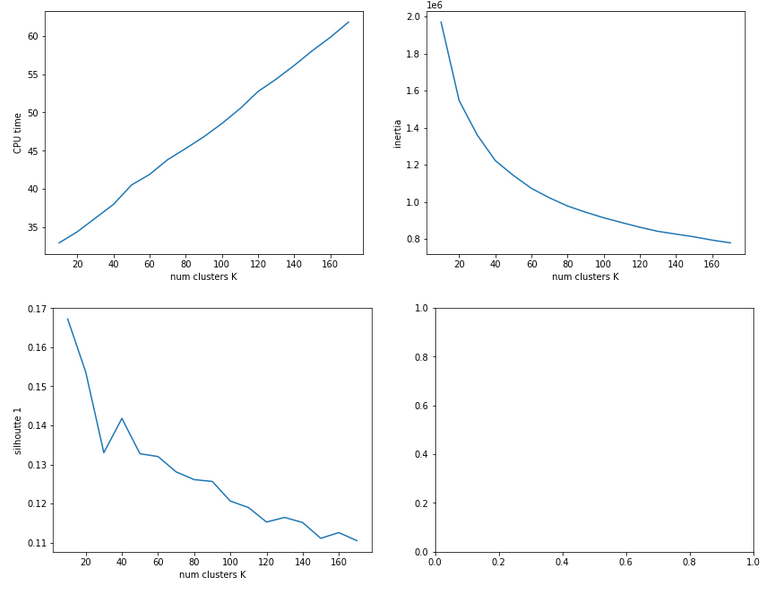The second run was executed with a higher resolution of K_(n+1) – K_n 5 = 5.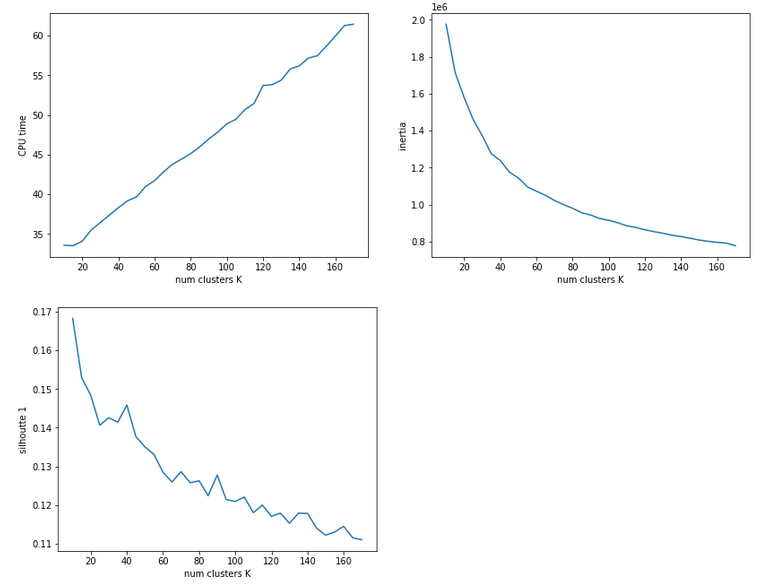We see that the CPU time to determine the centroids’ positions varies fairly linear with “K”. And even for 170 clusters it does not take more than a minute! So, CPU-time for cluster identification is not a major limitation.

Unfortunately, we do not see a clear elbow in the inertia curve! What you regard as a reasonable choice for the number K depends a lot on where you say the curve starts to flatten. You could say that this happens around K = 60 to 90. But the results for the silhouette-quantity indicate for our parameter setting that K=40, K=70, K=90 are interesting points. We shall look at these points a bit closer with higher resolution later on.

# Reduction of the regularization factor (for Ridge regularization)

Now, I want to discuss an important point which I did not find in the literature:
In my last article we saw that regularization plays a significant but also delicate role in reaching top accuracy values for the test dataset. We saw that Lambda2 = 0.2 was a good choice for a normalized input of the MNIST data. It corresponded to a certain ratio of the regularization term to average batch costs.
But when we reduce the number of input nodes we also reduce the number of total weights. So the weight values themselves will automatically become bigger if we want to get to similar good values at the second layer. But as the regularization term depends in a quadratic way on the weights we may assume that we roughly need a linear reduction of Lambda2. So, for K=100 clusters we may shrink Lambda2 to (0.2/784*100) = 0.025 instead of 0.2. In general:

Lambda2_cluster = Lambda2_std * K / (number of input nodes)

I applied this rule of a thumb successfully throughout experiments with clustering befor gradient descent.

# Reference run without clustering

We saw at the end of article XII that we could reach an accuracy of around 0.975 after 500 epochs under optimal circumstances. But in the case I presented ten I was extremely lucky with the statistical initial weight distribution and the batch composition. In other runs with the same parameter setup I got smaller accuracy values. So, let us take an ad hoc run with the following parameters and results:
Parameters: learn_rate = 0.001, decrease_rate = 0.00001, mom_rate = 0.00005, n_size_mini_batch = 500, n_epochs = 600, Lambda2 = 0.2, weights at
all layers in [-2*1.0/sqrt(num_nodes_layer), 2*1.0/sqrt(num_nodes_layer)]
Results: acc_train: 0.9949 , acc_test: 0.9735, convergence after ca. 550-600 epochs

The next plot shows (from left to right and the down) the evolution of the costs per batch, the averaged error of the last mini-batch during an epoch, the ratio of regularization to batch costs and the total costs of the training set, respectively .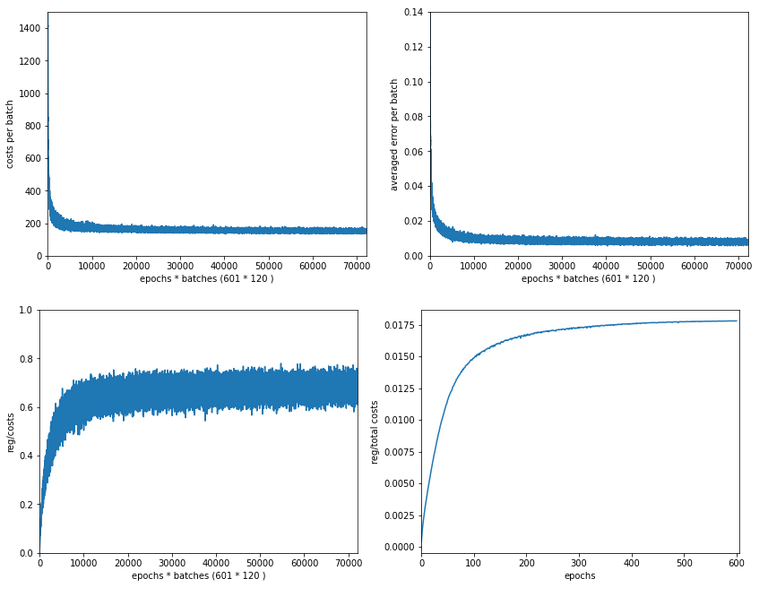The following plot summarizes the evolution of the total costs of the traaining set (including the regularization contribution) and the evolution of the accuracy on the training and the test data sets (in orange and blue, respectively).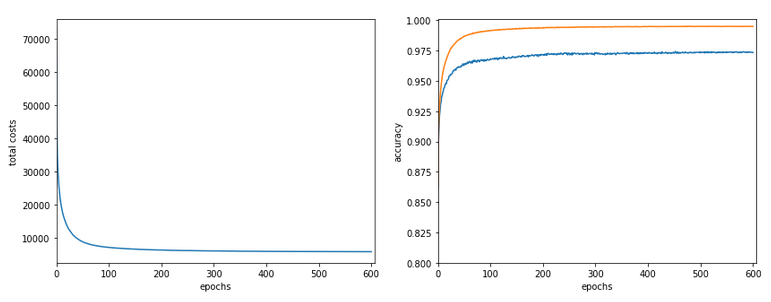The required computational time for the 600 epochs was roughly 18,2 minutes.

# Results of gradient descent based on a prior cluster identification

Before we go into a more detailed discussion of code adaption and test runs with things like clusters in unnormalized and normalized feature spaces, I want to show what we – without too much effort – can get out of using cluster detection ahead of gradient descent. The next plot shows the evolution of a run for K=70 clusters in combination with a special normalization: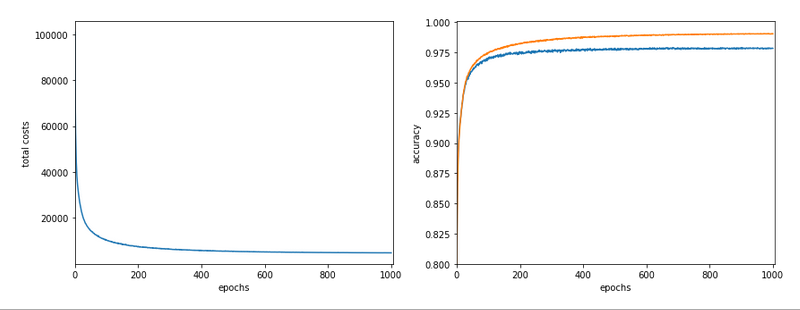and the total cost and accuracy evolution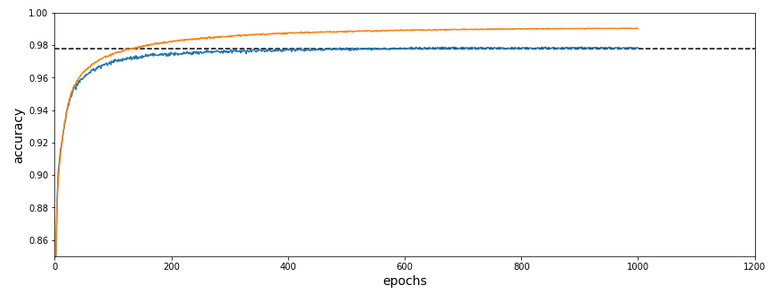The dotted line marks an accuracy of 97.8%! This is 0.5% bigger then our reference value of 97.3%. The total gain of %gt; 0.5% means however 18.5% of the remaining difference of 2.7% to 100% and we past a value of 97.8% already at epoch 600 of the run.

What were the required computational times?

If we just wanted 97.4% as accuracy we need around 150 epochs. And a total CPU time of 1.3 minutes to get to the same accuracy as our reference run. This is a factor of roughly 14 in required CPU time. For a stable 97.73% after epoch 350 we were still a factor of 5.6 better. For a stable accuracy beyond 97.8% we needed around 600 epochs – and still were by a factor of 3.3 faster than our reference run! So, clustering really brings some big advantages with it.

# Conclusion

In
this article I discussed the idea of introducing cluster identification in the (unnormalized or normalized) feature space ahead of gradient descent as a possible means to save computational time. A preliminary trial run showed that we indeed can become significantly faster by at least a factor of 3 up to 5 and even more. This is just due to the point that we reduced the number of input nodes and thus the number of mathematical calculations during matrix operations.

In the next article we shall have a more detailed look at clustering techniques in combination with normalization.

# A simple Python program for an ANN to cover the MNIST dataset – XIII – the impact of regularization

I continue with my growing series on a Multilayer perceptron and the MNIST dataset.

In the last article of the series we made some interesting experiences with the variation of the “leaning rate”. We also saw that a reasonable range for initial weight values should be chosen.

Even more fascinating was, however, the impact of a normalization of the input data on a smooth and fast gradient descent. We drew the conclusion that normalization is of major importance when we use the sigmoid function as the MLP’s activation function – especially for nodes in the first hidden layer and for input data which are on average relatively big. The reason for our concern were saturation effects of the sigmoid functions and other functions with a similar variation with their argument. In the meantime I have tried to make the importance of normalization even more plausible with the help of a a very minimalistic perceptron for which we can analyze saturation effects a bit more in depth; you get to the related article series via the following link:

There we also have a look at other normalizers or feature scalers.

But back to our series on a multi-layer perceptron. You may have have asked yourself in the meantime: Why did he not check the impact of the regularization? Indeed: We kept the parameter Lambda2 for the quadratic regularization term constant in all experiments so far: Lambda2 = 0.2. So, the question about the impact of regularization e.g. on accuracy is a good one.

# How big is the regularization term and how does it evolve during gradient decent training?

I add even one more question: How big is the relative contribution of the regularization term to the total loss or cost function? In our Python program for a MLP model we included a so called quadratic Ridge term:

Lambda2 * 0.5 * SUM[all weights**2], where bias nodes are excluded from the sum.

From various books on Machine Learning [ML] you just learn to choose the factor Lambda2 in the range between 0.01 and 0.1. But how big is the resulting term actually in comparison to the standard cost term, then, and how does the ratio between both terms evolve during gradient descent? What factors influence this ratio?

As we follow a training strategy based on mini-batches the regularization contribution was and is added up to the costs of each mini-batch. So its relative importance varies of course with the size of the mini-batches! Other factors which may also be of some importance – at least during the first epochs – could be the total number of weights in our network and the range of initial weight values.

Regarding the evolution during a converging gradient descent we know already that the total costs go down on the path to a cost minimum – whilst the weight values reach a stable level. So there is a (non-linear!) competition between the regularization term and the real costs of the “Log Loss” cost function! During convergence the relative importance of the regularization term may therefore become bigger until the ratio to the standard costs reaches an eventual constant level. But how dominant will the regularization term get in the end?

Let us do some experiments with the MNIST dataset again! We fix some common parameters and conditions for our test runs:
As we saw in the last article we should normalize the input data. So, all of our numerical experiments below (with the exception of the last one) are done with standardized input data (using Scikit-Learn’s StandardScaler). In addition initial weights are all set according to the sqrt(nodes)-rule for all layers in the interval [-0.5*sqrt(1/num_nodes), 0.5*sqrt(1/num_nodes)], with num_nodes meaning the number of nodes in a layer. Other parameters, which we keep constant, are:

Parameters: learn_rate = 0.001, decrease_rate = 0.00001, mom_rate = 0.00005, n_size_mini_batch = 500, n_epochs = 800.

I added some statements to the method for cost calculation in order to save the relative part of the regularization terms with respect to the total costs of each mini-batch in a Numpy array and plot the evolution in the end. The changes are so simple that I omit showing the modified code.

# A first look at the evolution of the relative contribution of regularization to the total loss of a mini-batch

How does the outcome of gradient descent look for standardized input data and a Lambda2-value of 0.1?

Lambda2 = 0.1
Results: acc_train: 0.999 , acc_test: 0.9714, convergence after ca. 600 epochs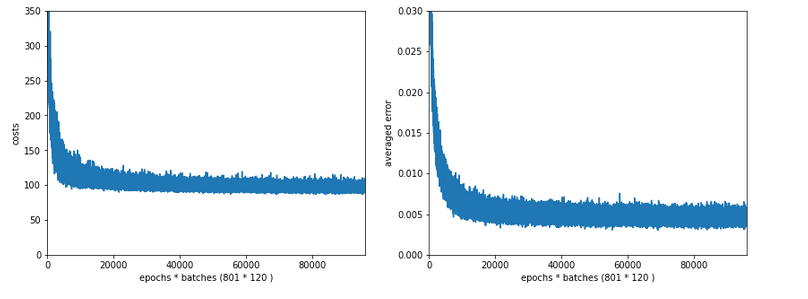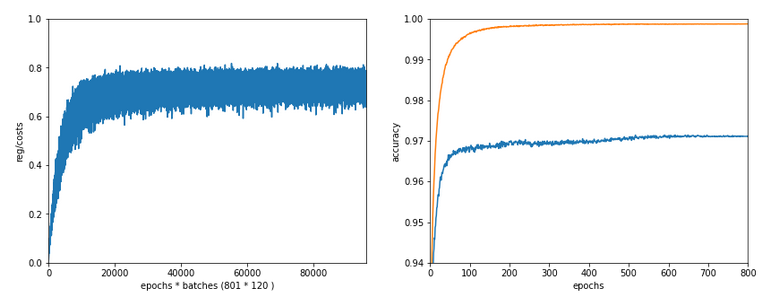We see that the regularization term actually dominates the total loss of a mini-batch at convergence. At least with our present parameter setting. In comparisoin to the total loss of the full training set the contribution is of course much smaller and typically below 1%.

# A small Lambda term

Let us reduce the regularization term via setting Lambda = 0.01. We expect its initial contribution to the costs of a batch to be smaller then, but this does NOT mean that the ratio to the standard costs of the batch automatically shrinks significantly, too:

Lambda2 = 0.01
Results: acc_train: 1.0 , acc_test: 0.9656, convergence after ca. 350 epochs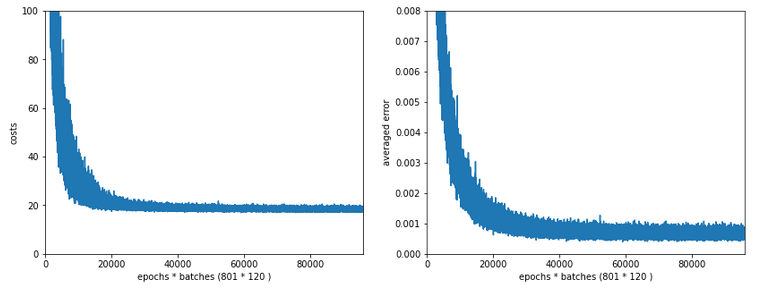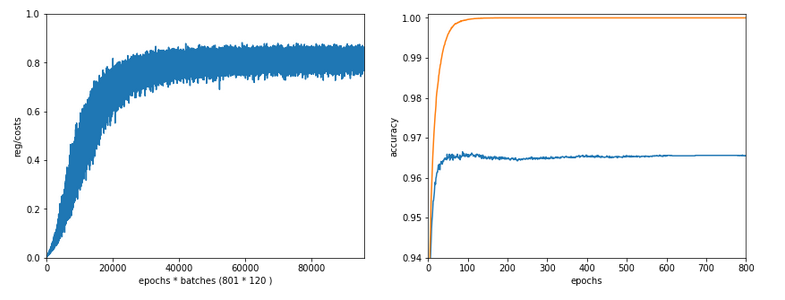Note the absolute scale of the costs in the plots! We ended up at a much lower level of the total loss of a batch! But the relative dominance of regularization at the point of convergence actually increased! However, this did not help us with the accuracy of our MLP-algorithm on the test data set – although we perfectly fit the training set by a 100% accuracy.

In the end this is what regularization is all about. We do not want a total overfitting, a perfect adaption of the grid to the training set. It will not help in the sense of getting a better general accuracy on other input data. A Lambda2 of 0.01 is much too small in our case!

# Slightly bigger regularization with Lambda2 = 0.2

So lets enlarge Lambda2 a bit:
Lambda2 = 0.2
Results: acc_train: 0.9946 , acc_test: 0.9728, convergence after ca. 700 epochs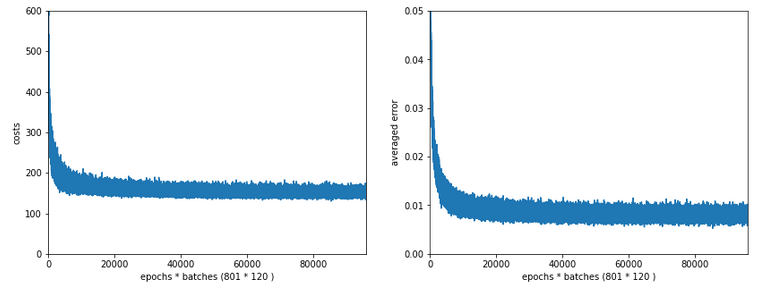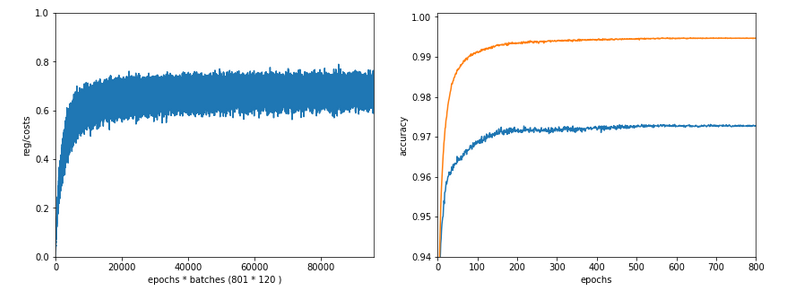We get an improved accuracy!

# Two other cases with significantly bigger Lambda2

Lambda2 = 0.4
Results: acc_train: 0.9858 , acc_test: 0.9693, convergence after ca. 600 epochs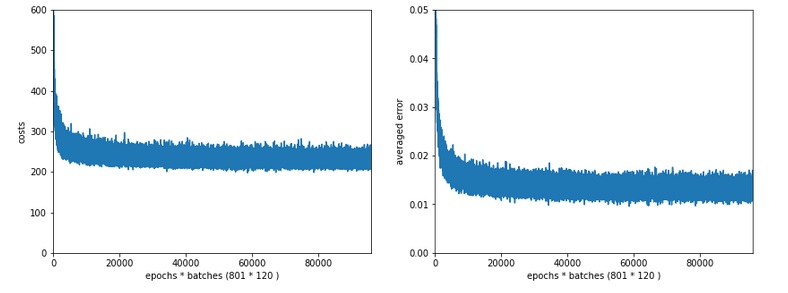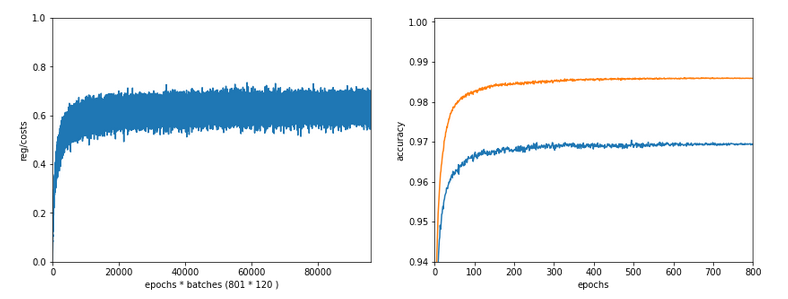Lambda2 = 0.8
Results: acc_train: 0.9705 , acc_test: 0.9588, convergence after ca. 400 epochs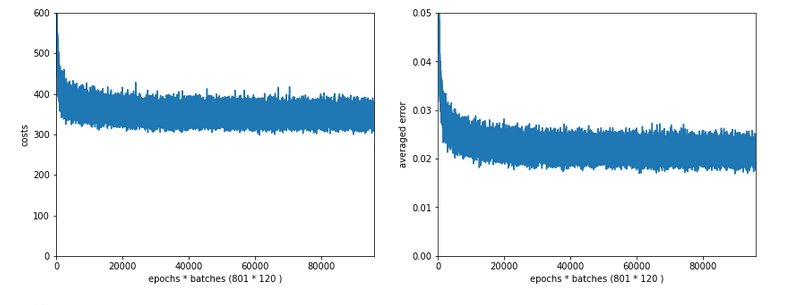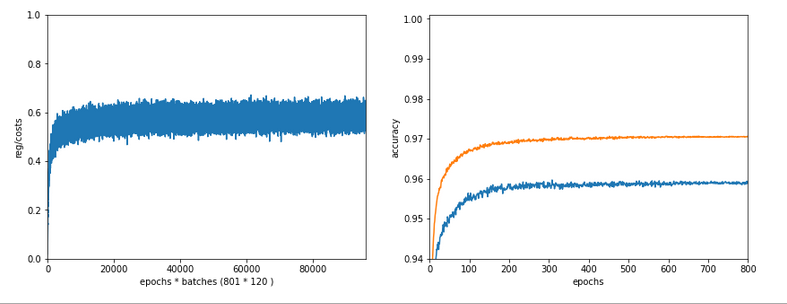OK, but in both cases we see a significant and systematic trend towards reduced accuracy values on the test data set with growing Lambda2-values > 0.2 for our chosen mini-batch size (500 samples).

# Conclusion

We learned a bit about the impact of regularization today. Whatever the exact Lambda2-value – in the end the contribution of a regularization term becomes a significant part of the total loss of a mini-batch when we approached the total cost minimum. However, the factor Lambda2 must be chosen with a reasonable size to get an impact of regularization on the final minimum position in the weight-space! But then it will help to improve accuracy on general input data in comparison to overfitted solutions!

But we also saw that there is some balance to take care of: For an optimum of generalization AND accuracy you should neither make Lambda2 too small nor too big. In our case Lambda2 = 0.2 seems to be a reasonable and good choice. Might be different with other datasets.

All in all studying the impact of a variation of achieved accuracy with the factor for a Ridge regularization term seems to be a good investment of time in ML projects. We shall come back to this point already in the next articles of this series.

In the next article

A simple Python program for an ANN to cover the MNIST dataset – XIV – cluster detection in feature space

we shall start to work on cluster detection in the feature space of the MNIST data before using gradient descent.